Disclosure: This post may contain affiliate links, meaning when you click the links and make a purchase, we receive a commission.

# What is the Order & Precedence of Operations in Excel?

Understanding the order and precedence of operations in mathematical formulas or in an Excel Formula is very important. Excel has total 17 operators that you can use them to build complex Excel formulas.

Here is the list of operators and their precedence: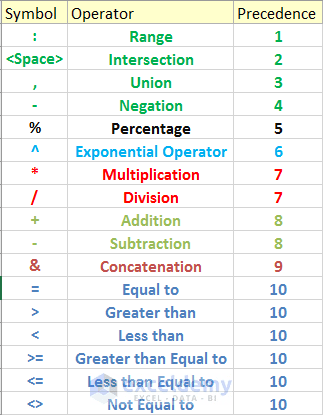Range operator, intersection operator (it is actually Space) and union operator as a whole known as reference Operators.

Which operator will be evaluated first depends on the precedence of the operator. Look at the operator precedence list (above image).

## Order & Precedence of Operations

### Range Operator (Precedence Level 1)

The first one is the Range operator.

Let’s explain this with an Excel formula. Select cell J15, and click on the formula bar.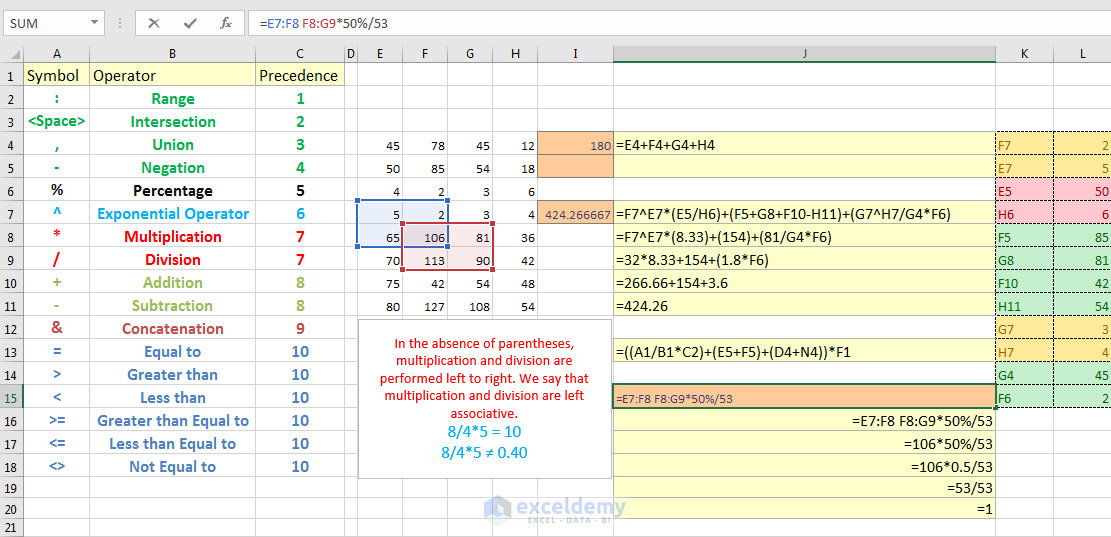In this formula, E7 colon F8 refer to the blue range and F8 colon G9 refer to the red range. So, in this formula, these two ranges (E7: F8 and F8: G9) will be evaluated at first.

### Space Operator (Precedence Level 2)

Then the Space operator. Its precedence level is 2. So, this whole part (E7:F8 F8:G9) will generate the Intersection of the blue range and the red range.

Intersection means the common part. The common part of the blue range and the red range is 106.

### Union Operator (Precedence Level 3)

The next operator in the precedence level is the union operator, the comma.

If I put comma in this formula between these ranges and delete the space (the formula will be like this: E7:F8, F8:G9), then this whole formula will refer to these cells, cells that have values 5, 2, 65, 106, 81, 113, and 90; the combination of the two ranges. This Union operator has the Precedence level of 3.

### Negation operator (Precedence Level 4)

Then Negation operator. Its precedence level is 4. Negation operator is used to negating a single number, it is not subtraction; subtraction works on two numbers.

### Percentage Operator (Precedence Level 5)

Then the percentage operator. Its precedence level is 5.

### Exponential Operator (Precedence Level 6)

The next one is the Exponential operator, the Caret sign. Its precedence level is 6.

### Multiplication and Division Operators (Both have Precedence Level 7)

Multiplication and Division have the same precedence level (7). They are calculated after the exponential operator. When operators have same precedence level, they are evaluated from left to right.

Just look at this example.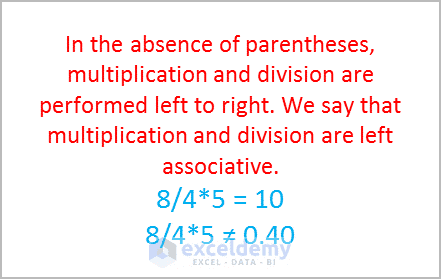It is 8 divided by 4, then multiplied by 5. Which calculation will be evaluated first? Is it 8 divided by 4 or 4 times 5. At this point, remember that multiplication and division are left associative. It means evaluation will happen from left to right. So, 8 will be divided by 4 at first, then the result 2 will be multiplied by 5. So, the ultimate result is 10. If you multiply 4 and 5 first, then divide 8 by the result 20, you will get the wrong answer.

### Plus, Minus Operators (Precedence Level 8)

The next two operators plus and minus have same precedence level 8. They are evaluated after multiplication and division.

### Ampersand Operator (Precedence Level 9)

After Plus and Minus, ampersand operator is evaluated. Its precedence level is 9.

### Logical Operators (Precedence Level 10)

Then comes the logical operators. These six logical operators have the same precedence level, the level is 10. They are evaluated at the end of the calculation. So, you have got an idea about precedence level. It is very important to understand the Precedence Level of Operators.

## Controlling Precedence Level using Parentheses

You can control the Precedence level using the Parenthesis or simply you can call it bracket. Expressions within parentheses are always calculated first. Let’s use the precedence level idea in some practical examples.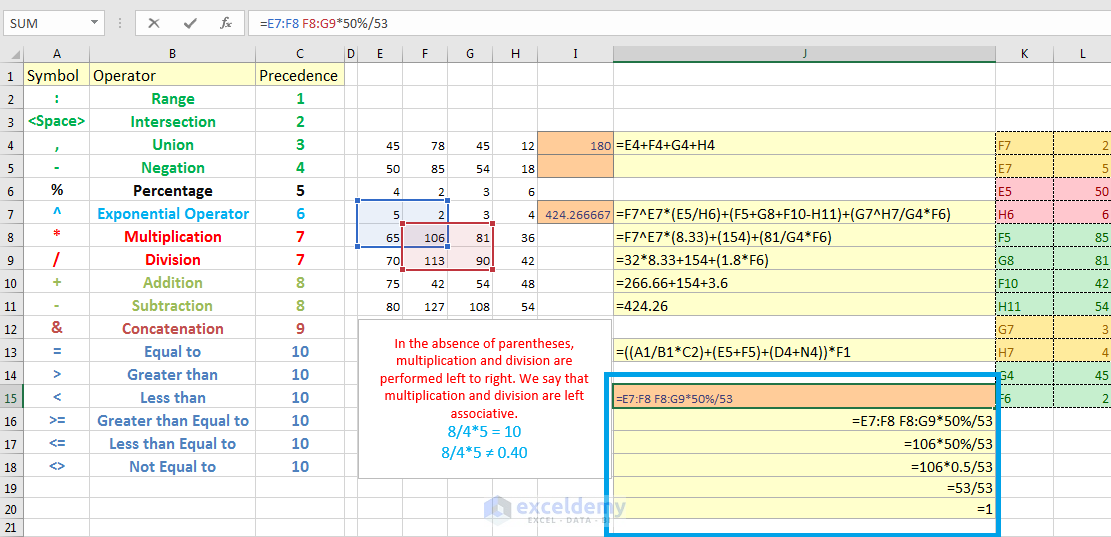Let’s work with this formula at first (formula in cell J15).

Click on the Formula bar, in this formula, Excel will work the Range Operators at first. As Range Operator has the Highest Precedence level. The intersection of these two ranges is 106. So, the formula looks like this internally (in cell J17).

=106*50%/53

At this stage of the formula, 50% will be evaluated now, 50% means actually point 5 (0.5). The formula will be like this one now internally (in cell J18).

=106*0.5/53

There are two operators now: multiplication and division. They have same precedence level. You know when operators have same precedence level, they are calculated from left to right. So, this part (106*0.5) will be evaluated first.

Now the formula is 53/53 that results in 1.

So, this is how precedence level works in an Excel formula.

## A simple example

Now, I select cell I4, you see this cell has a formula.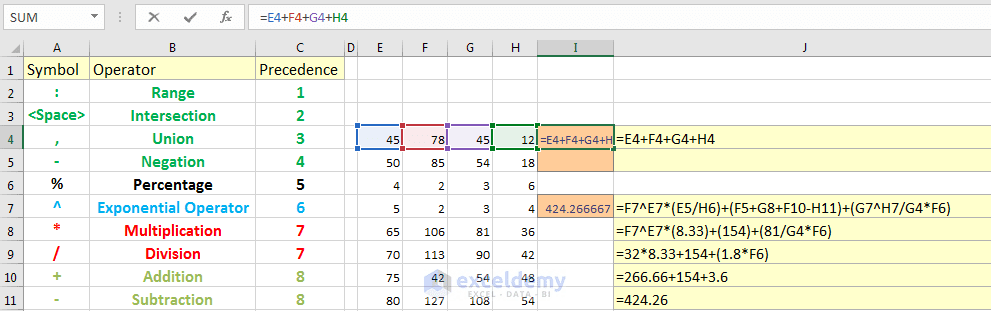The formula is showed on the right of the cell. It is a very straightforward formula. It’s just adding cell E4, F4, G4, and H4.

In real life, a formula is not always straight forward like this one.

Let’s show you how can do a mistake. Say you want to add cell E4, F4, G4, and H4, then you want to multiply the result with cell E7. Mistakenly, you write the formula like this one:

=E4+F4+G4+H4*E7

You thought that the result would be 900.

As you already calculated that the sum of the cells E4, F4, G4, and H4 is 180. So, when it will be multiplied by 5, the result would be 900. If you press return, you will get a result that you did not expect.

Why this fault?

Let’s observe the formula. According to the precedence level, cell H4 and cell E7 are multiplied first, then the result of the multiplication is added to cells E4, F4, and G4.

If you want to add these cells (E4, F4, G4 and H4) at first, then you have to use parentheses in the expression.

Just edit the formula in the following way, and you will get the result as expected (900).

=(E4+F4+G4+H4)*E7 = 900

## Another complex example

To make the idea clearer, I have made a slightly complex formula. The formula is applied to the cell I7, and the formula is showed in the cell J7.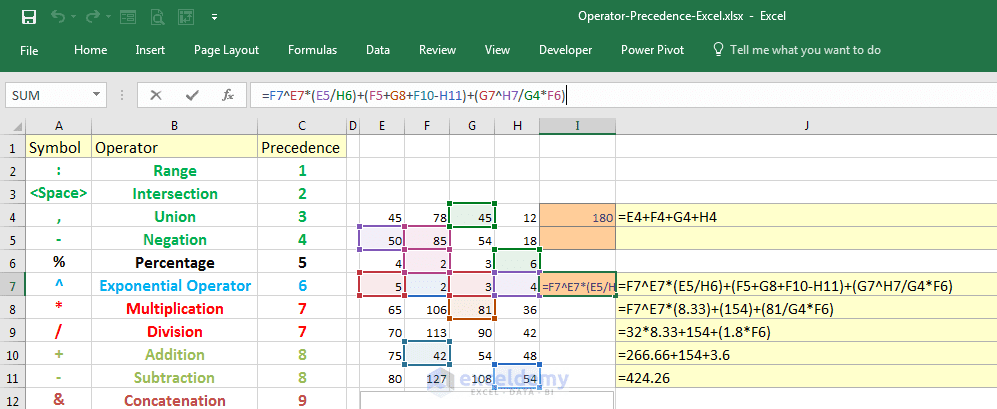I have used 5 types of operators in this formula.

1. Exponent
2. Multiplication
3. Division
4. Plus
5. Minus

Parentheses are also used in this expression. So according to precedence level, you will evaluate the expressions within the parentheses at first. So, this expression (E5/H6), this expression (F5+G8+F10-H11) and this expression (G7^H7/G4*F6) will be evaluated at first.

• (E5/H6) results in 8.33
• (F5+G8+F10-H11) results in 154
• And in this expression (G7^H7/G4*F6), G7 to the power H7 will be calculated at first. The reason is exponentiation has higher precedence level over multiplication and division. G7 to the power H7 results in 81.

So, the new expression is like this one.

=F7^E7*(8.33) + (154) + (81/G4*F6)

Now F7 to the power E7 is equal to 32, 154 will be as it is, and in this expression according to left associativity, 81 divided by G4 is equal to 1.8. New expression will be like this one.

=32*8.33 + 154 + (1.8*F6)

Now the evaluation is simple. At this expression, multiplications will be calculated at first, the results are 266.66 (32*8.33) plus, 154 plus 3.6. So, the ultimate result is 424.26.

## More complex formula

With the above example, I have shown you an interesting way to work with the complex formula. Let’s look at a complex excel formula.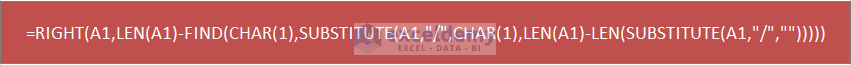A formula like this one may frighten you, but believe me, if you break down this formula in my above-mentioned way, this is a very simple formula.

## Nested Parentheses

The next thing, I am going to discuss is about nested parentheses.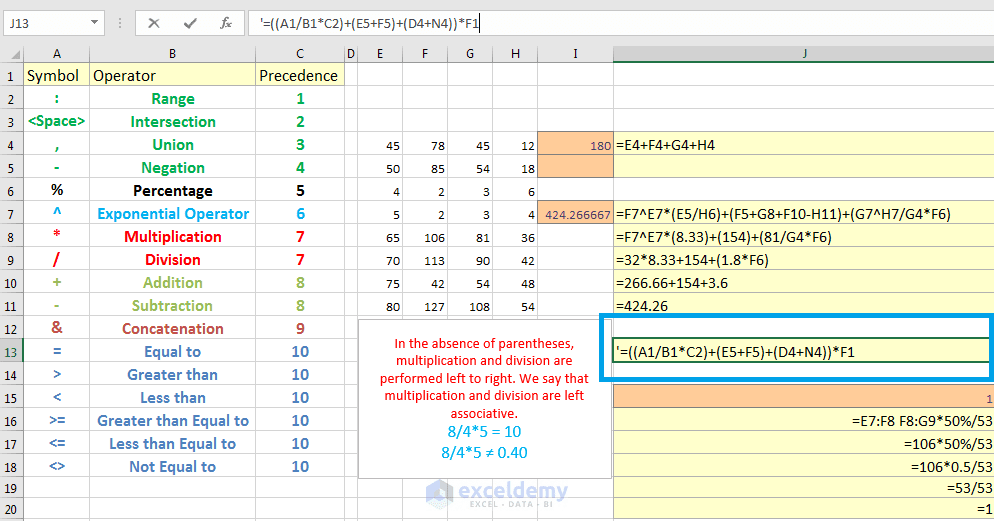Look at this hypothetical example; you see these three parentheses are within one parenthesis. In these type of cases, Excel will handle at first the deepest parentheses.

## If you open a parenthesis, close it

If you open a parenthesis, then you have to close it. If Excel gets a mismatched parenthesis, then its shows a message with corrected formula. Say in the cell I8, I type an equal sign, then opening parenthesis, select cell E8, plus sign, then select cell F8. I don’t close the parenthesis, just press Return. You see excel shows a message with the corrected formula, if you accept the correction, then click Yes, if you don’t accept the correction, click No.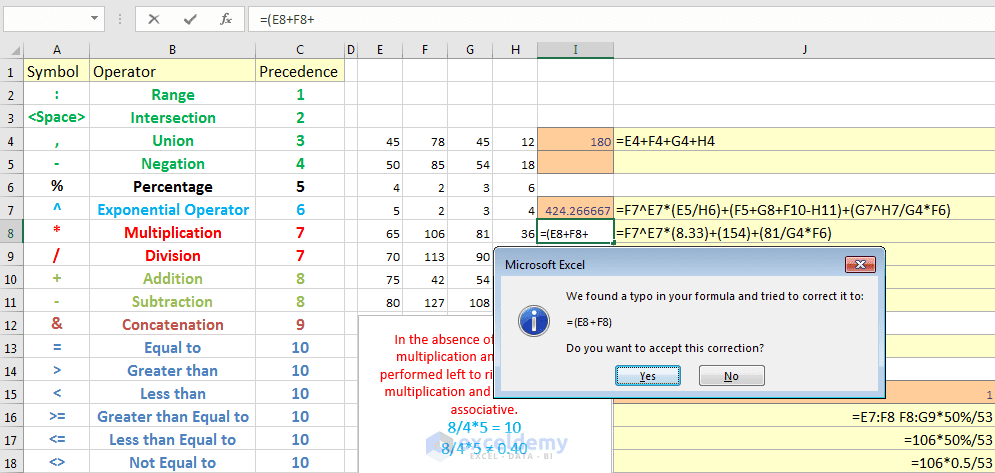So, this is how you can use operator precedence in the Excel formulas.

Operator-Precedence-Excel.xlsx

Hello!
Welcome to my Excel blog! It took me some time to be a fan of Excel. But now I am a die-hard fan of MS Excel. I learn new ways of doing things with Excel and share here. Not only how to guide on Excel, but you will get also topics on Finance, Statistics, Data Analysis, and BI. Stay tuned!

1. ReplyPrabhudeva GD January 14, 2017 at 10:26 PM

Best Notes with examples, thank you sir

• ReplyKawser January 15, 2017 at 1:23 PM

Thanks for the feedback, Prabhudeva!

2. ReplyA Venugopalarao November 30, 2018 at 12:23 PM

Best explanation, Thanque sir